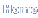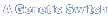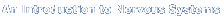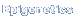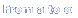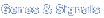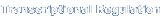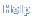Choose a Title A Genetic Switch Intro to Nervous Systems Epigenetics From a to alpha Genes and Signals Transcriptional Regulation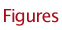Click on the highlighted text below to see the Chapter Figures

Transcriptional Regulation in Eukaryotes: Concepts, Strategies, and Techniques, Second Edition

Chapter 01 Figures
Figure 01
Figure 02
Figure 03
Figure 04
Figure 05
Figure 06
Figure 07
Figure 08
Figure 09
Figure 10
Figure 11
Figure 12
Figure 13
Figure 14
Figure 15

Chapter 02 Figures
Figure 01
Figure 02
Figure 03

Chapter 03 Figures
Figure 01
Figure 02
Figure 03
Figure 04
Figure 05
Figure 06
Figure 07

Chapter 04 Figures
Figure 01
Figure 02
Figure 03
Figure 04
Figure 05
Figure 06
Figure 07
Figure 08

Chapter 05 Figures
Figure 01
Figure 01
Figure 01
Figure 02
Figure 03
Figure 04
Figure 05
Figure 06
Figure 07

Chapter 06 Figures
Figure 01
Figure 02
Figure 03
Figure 04
Figure 05
Figure 06
Figure 07

Chapter 07 Figures
Figure 01
Figure 02
Figure 03
Figure 04
Figure 05
Figure 06
Figure 07
Figure 08

Chapter 08 Figures
Figure 01
Figure 02
Figure 03
Figure 04
Figure 05
Figure 06
Figure 07
Figure 08
Figure 09

Chapter 09 Figures
Figure 01
Figure 02
Figure 03
Figure 04
Figure 05
Figure 06
Figure 07
Figure 08
Figure 09
Figure 10
Figure 11
Figure 12

Chapter 10 Figures
Figure 01
Figure 02
Figure 03
Figure 04
Figure 05
Figure 06
Figure 07
Figure 08

Chapter 11 Figures
Figure 01
Figure 02
Figure 03
Figure 04
Figure 05
Figure 06
Figure 07
Figure 08
Figure 09
Figure 10
Figure 11
Figure 12
Figure 13
Figure 14
Figure 15
Figure 16
Figure 17
Figure 18
Figure 19
Figure 20

Chapter 12 Figures
Figure 01
Figure 02
Figure 03
Figure 04
Figure 05
Figure 06
Figure 07
Figure 08
Figure 09
Figure 10
Figure 11
Figure 12
Figure 13
Figure 14
Figure 15
Figure 16
Figure 17
Figure 18

Chapter 13 Figures
Figure 01
Figure 02
Figure 03
Figure 04
Figure 05
Figure 06
Figure 07
Figure 08
Figure 09

 © 2009 by Cold Spring Harbor Laboratory Press. All rights reserved.No part of these pages, either text or image, may be used for any purpose other than personal use. Therefore, reproduction, modification, storage in a retrieval system, or retransmission, in any form or by any means, electronic, mechanical, or otherwise, for reasons other than personal use, is strictly prohibited without prior written permission.